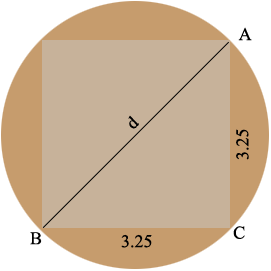SEARCH HOMEMath Central Quandaries & QueriesQuestion from Bryan: I want to know what the smallest diameter round is that will make a 3-1/4" square? Is there a formula for that? I am milling round stock into square. Thank you.Hi Bryan,

The key to solving this is Pythagoras Theorem. It amazes me how often the key to solving a practical problem is Pythagoras Theorem.Suppose the diameter of the round stock is $d$ inches. In the diagram above the triangle $ABC$ is a right triangle and Pythagoras Theorem states that for a right triangle the square of the length of the hypotenuse (the long side) is the sum of the squares of the lengths of the other two sides. Thus

$d^2 = 3.25^2 + 3.25^ 2 = 10.5625 + 10.5625 = 21.125 \mbox{ square inches}$

and thus

$d = \sqrt{21.125} = 4.596 \mbox{ inches}$

I hope this helps,
HarleyMath Central is supported by the University of Regina and The Pacific Institute for the Mathematical Sciences.